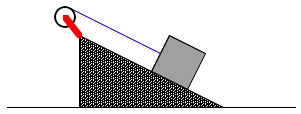1.     In tightening cylinder-head bolts in an automobile engine, the critical quantity is the torque applied to the bolts. Why is this more important than the actual force applied to the wrench handle?

2.     Consider a yo-yo with its string wound up, ready for action.  Suppose that we know nothing about this yo-yo except that it has the shape of a thin cylinder, with the string wrapped around the outside of the cylinder.  (So this is even simpler than a regular yo-yo.)  If you release the yo-yo from rest, the string will unwind and it will accelerate downward, but at what rate?

(a)   Make a free-body diagram and choose a convenient coordinate system for the yo-yo as it falls.

(b)  Apply Newton's 2nd Law (SF = ma) to your FBD.  Assuming that the yo-yo’s mass and radius are known quantities, can you determine the acceleration of the yo-yo in terms of known quantities from this equation?  Why or why not?

(c)   Now choose a rotational coordinate system and apply the torque equation to the yo-yo (the moment of inertia of a disk is MR2).

(d)  What is the relationship between a (the angular acceleration of the yo-yo) and a (the linear acceleration of the yo-yo)?

(e)   Using the results of (b), (c) and (d), determine the acceleration of the yo-yo.  Does the answer depend on the mass or radius of the yo-yo?  What does it depend on?

(f)   What is the tension in the string as the yo-yo falls?  Give your answer as some fraction or multiple of the weight of the yo-yo.

3.     Below is a figure that shows three identical yo-yos initially at rest on a horizontal surface.  For each yo-yo the string is pulled in the direction shown.  In each case there is sufficient friction for the yo-yo to roll without slipping.  Split into three groups.  Each group should draw the free-body diagram for one of the yo-yo's and convince themselves and the other two groups that they know the direction the chosen yo-yo will rotate.  If someone in the group reads this ahead of time and brings a yo-yo to workshop it might help!4.     A block with mass m=5.00 kg slides down a surface inclined 36.9 degrees to the horizontal (see the figure below).  The coefficient of kinetic friction is 0.25.  A string attached to the block is wrapped around a flywheel on a fixed axis through its center.  The flywheel has mass 20.0 kg, radius R = 0.200 m, and a moment of inertia with respect to the axis 0.400 kg-m2.

(a)   What is the acceleration of the block down the plane?

(b)  What is the tension in the string?